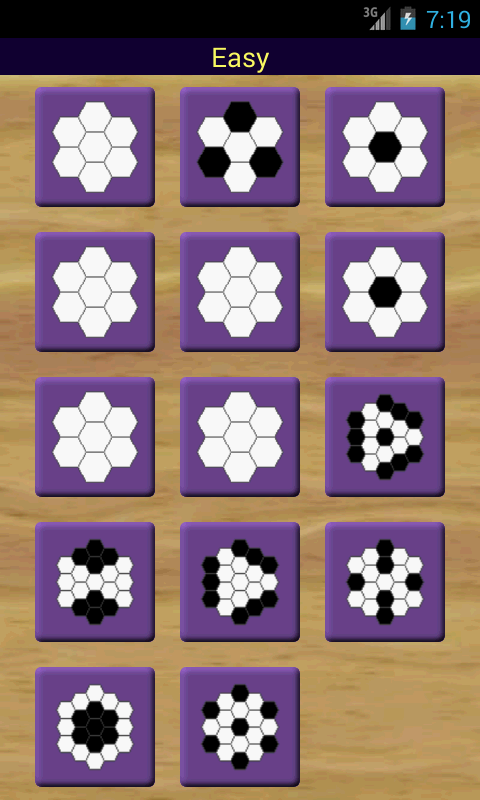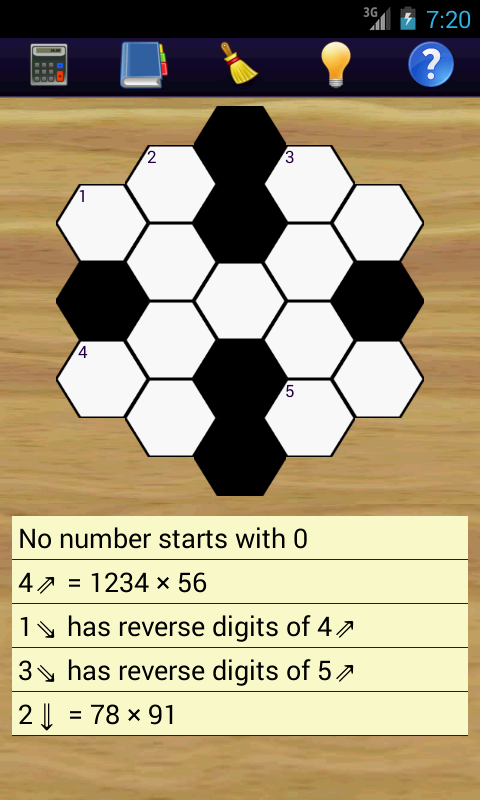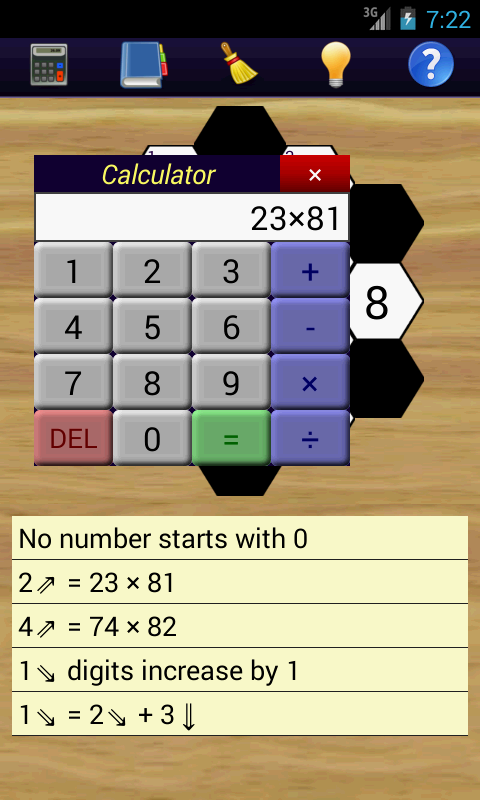Math Hexagon Puzzles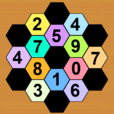Math Hexagon Puzzles is a free set of cross-figure logic puzzles (the numerical version of a crossword)

#### Using Hexagons

The puzzles us a hexagonal grid instead of the usual crossword puzzle grid. Instead of having clues for across and down, the clues go diagonally up, diagonally down and down instead.

#### Solving the Puzzles

Clues are given to each puzzle and the player fills in blank spaces with numbers making sure they meet the criteria of the clues. There are 4 different sets of puzzles ranging from Easy puzzles, designed to help ease players into the process of completing the math puzzles, and finishing with Very Hard puzzles which require more time to think through and solve. The player has to achieve a certain number of puzzles before they can unlock later sets of puzzles.

Each puzzle has clues, calculator, list of square numbers, list of cubed numbers and a list of prime numbers to assist the player in completion of the puzzles. The player also starts with 5 hints and receives extra ones when a level is completed to help solve the puzzles which are causing a challenge.

There are a total of 60 puzzles with four levels of difficulty, Easy, Normal, Hard and Very Hard

#### Difficulty Stages

• Easy: 14 puzzles
• Normal: 22 puzzles
• Hard: 12 puzzles
• Very Hard: 12 puzzles

Version 1 is the latest major version of Math Hexagon Puzzles.

#### Images

 Book of Lists Puzzle in Progress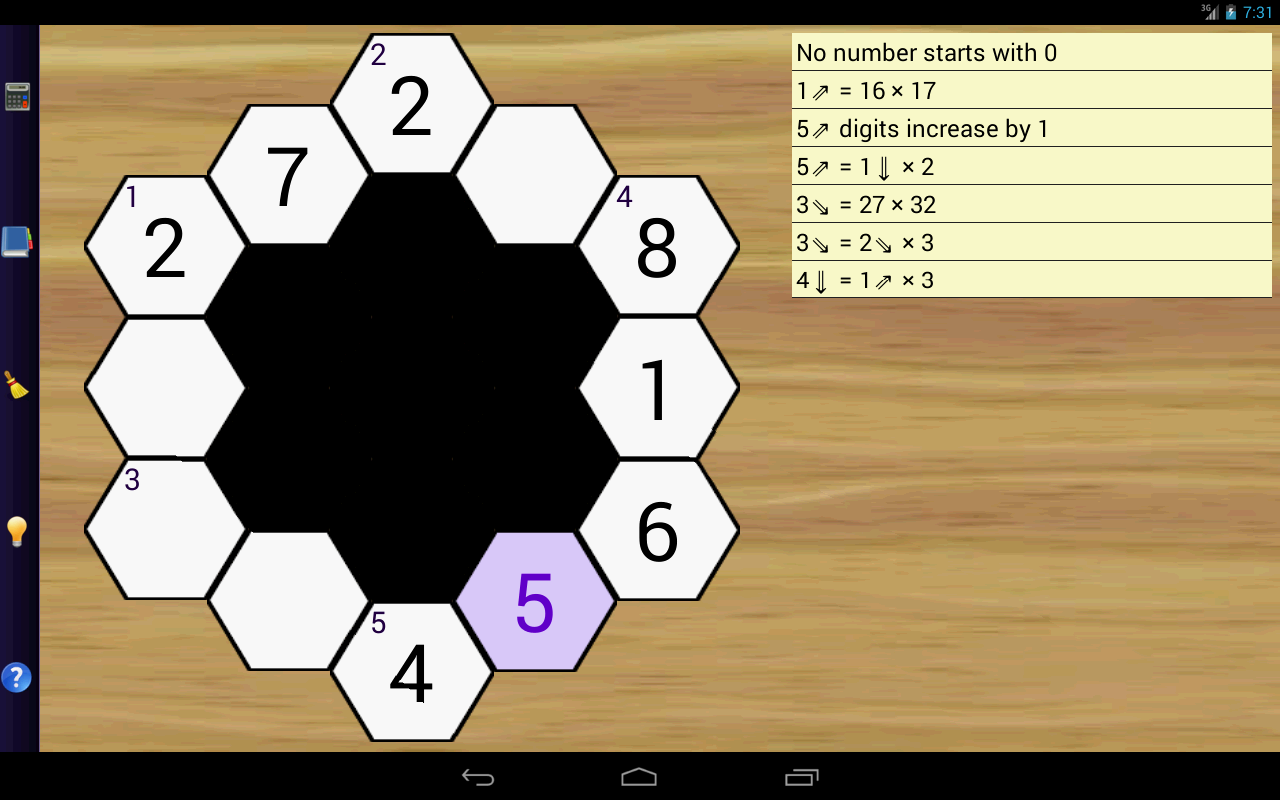Beginner Puzzles New Puzzle Calculator